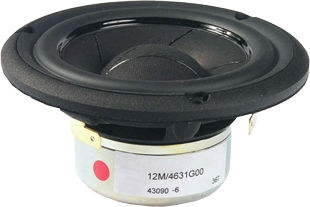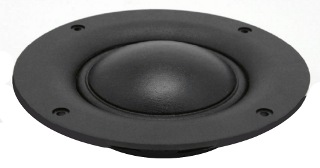ASE Tweeter Fullrange Mids Woofer Subwoofer Car-HiFi no longer available

# ScanSpeak

Founded in 1970, Scan-Speak still resides at its original address in Videbaek, Denmark. Located in the heart of the Jutland peninsula, the acoustic engineers adhere to the legendary policy of "Never compromise".

## Mids## Illuminatoropen a bigger photo

recommended cabinet 1:
closed cabinet with 0,5 L volume
from 203/128 Hz (-3dB/-8dB)

recommended cabinet 2:
1,2 L volume bass reflex cabinet
with HP35 reflex tube, 18 cm long.
from 121/91 Hz (-3dB/-8dB).

## ScanSpeak 12MU/4731T00

order no. ss-12MU-4731T

EUR 288,07excl. VAT: € 242.08 / \$ 268.70

11 cm bass-midrange driver with high quality cone. The magnet system is based on Neodymium technology.

• power handling (continuous/programme) = 80/150 W
• frequency range = 70-6000 Hz
• resonance frequency fs = 64 Hz
• impedance R = 4 Ohm
• sound pressure level SPL = 90 dB (2,83V; 1m)
• DC resistance Re = 3,1 Ohm
• force factor BL = 5,1 N/A
• voice coil inductance L = 0,11 mH
• effective piston radiating area Sd = 58 cm2
• effective mechanical mass incl. air load mms = 5,4 g
• equivalent volume of compliance Vas = 5,4 l
• total Q factor Qts = 0,24 (Qms=3,64, Qes=0,26)
• voice coil diameter = 32 mm
• maximum peak linear excursion vibration xlin = +/- 3,5 mm
• mounting diameter d = 101 mm
• overall diameter d = 120 mm
• mounting depth (not countersunk) t = 70 mm
PDF data sheetopen a bigger photo

recommended cabinet 1:
closed cabinet with 0,74 L volume
from 181/114 Hz (-3dB/-8dB)

recommended cabinet 2:
2,1 L volume bass reflex cabinet
with HP35 reflex tube, 12 cm long.
from 102/78 Hz (-3dB/-8dB).

## ScanSpeak 12MU/8731T00

order no. ss-12MU-8731T

EUR 288,07excl. VAT: € 242.08 / \$ 268.70

11 cm bass-midrange driver with high quality cone. The magnet system is based on Neodymium technology.

• power handling (continuous/programme) = 80/150 W
• frequency range = 70-6000 Hz
• resonance frequency fs = 66 Hz
• impedance R = 8 Ohm
• sound pressure level SPL = 87,2 dB (2,83V; 1m)
• DC resistance Re = 5,9 Ohm
• force factor BL = 6,1 N/A
• voice coil inductance L = 0,13 mH
• effective piston radiating area Sd = 58 cm2
• effective mechanical mass incl. air load mms = 4,7 g
• equivalent volume of compliance Vas = 5,8 l
• total Q factor Qts = 0,29 (Qms=3,63, Qes=0,31)
• voice coil diameter = 32 mm
• maximum peak linear excursion vibration xlin = +/- 3,4 mm
• mounting diameter d = 101 mm
• overall diameter d = 120 mm
• mounting depth (not countersunk) t = 70 mm
PDF data sheet

## Revelatoropen a bigger photo

recommended cabinet 1:
closed cabinet with 0,42 L volume
from 177/111 Hz (-3dB/-8dB)

recommended cabinet 2:
1,4 L volume bass reflex cabinet
with HP35 reflex tube, 21 cm long.
from 93/73 Hz (-3dB/-8dB).

## ScanSpeak 12M/4631G00

order no. ss-12M-4631G

EUR 279,00excl. VAT: € 234.45 / \$ 260.24

10 cm bass-midrange driver with paper cone. The magnet system is based on Neodymium technology.

• power handling (continuous/programme) = 40 W
• frequency range = 75-8000 Hz
• resonance frequency fs = 75 Hz
• impedance R = 4 Ohm
• sound pressure level SPL = 89 dB (2,83V; 1m)
• DC resistance Re = 3,2 Ohm
• force factor BL = 5,3 N/A
• voice coil inductance L = 0,22 mH
• effective piston radiating area Sd = 49 cm2
• effective mechanical mass incl. air load mms = 6,5 g
• equivalent volume of compliance Vas = 2,3 l
• total Q factor Qts = 0,33 (Qms=5,57, Qes=0,35)
• voice coil diameter = 38 mm
• maximum peak linear excursion vibration xlin = +/- 3 mm
• mounting diameter d = 92 mm
• overall diameter d = 115,5 mm
• mounting depth (not countersunk) t = 51,4 mm
PDF data sheetopen a bigger photo

recommended cabinet 1:
closed cabinet with 1,8 L volume
from 120/76 Hz (-3dB/-8dB)

recommended cabinet 2:
4,1 L volume bass reflex cabinet
with HP35 reflex tube, 15 cm long.
from 74/55 Hz (-3dB/-8dB).

## ScanSpeak 15M/4531K00

order no. ss-15M-4531K00

EUR 196,46excl. VAT: € 165.09 / \$ 183.25

14 cm bass-midrange driver with paper cone.

• power handling (continuous/programme) = 50/150 W
• frequency range = 40-2500 Hz
• resonance frequency fs = 35 Hz
• impedance R = 4 Ohm
• sound pressure level SPL = 90 dB (2,83V; 1m)
• DC resistance Re = 3,5 Ohm
• force factor BL = 6 N/A
• voice coil inductance L = 0,17 mH
• effective piston radiating area Sd = 95 cm2
• effective mechanical mass incl. air load mms = 11 g
• equivalent volume of compliance Vas = 23,8 l
• total Q factor Qts = 0,23 (Qms=4,8, Qes=0,24)
• voice coil diameter = 38 mm
• maximum peak linear excursion vibration xlin = +/- 3 mm
• mounting diameter d = 125 mm
• overall diameter d = 148 mm
• mounting depth (not countersunk) t = 76,7 mm
PDF data sheetopen a bigger photo

## ScanSpeak 18M/4631T00

order no. ss-18m4631t00

EUR 215,00excl. VAT: € 180.67 / \$ 200.55

17 cm bass-midrange driver with paper cone.

• power handling (continuous/programme) = 120/250 W
• frequency range = 60-7000 Hz
• resonance frequency fs = 65 Hz
• impedance R = 4 Ohm
• sound pressure level SPL = 92 dB (2,83V; 1m)
• DC resistance Re = 3,5 Ohm
• force factor BL = 5,6 N/A
• voice coil inductance L = 0,16 mH
• effective piston radiating area Sd = 154 cm2
• effective mechanical mass incl. air load mms = 14,3 g
• equivalent volume of compliance Vas = 14 l
• total Q factor Qts = 0,6 (Qms=5,65, Qes=0,67)
• voice coil diameter = 38 mm
• maximum peak linear excursion vibration xlin = +/- 3 mm
• mounting diameter d = 156 mm
• overall diameter d = 182 mm
• mounting depth (not countersunk) t = 84,9 mm
PDF data sheetopen a bigger photo

## ScanSpeak 18M/8631T00

order no. ss-18M8631T00

EUR 215,00excl. VAT: € 180.67 / \$ 200.55

17 cm bass-midrange driver with paper cone.

• power handling (continuous/programme) = 120/250 W
• frequency range = 60-7000 Hz
• resonance frequency fs = 65 Hz
• impedance R = 8 Ohm
• sound pressure level SPL = 89 dB (2,83V; 1m)
• DC resistance Re = 6 Ohm
• force factor BL = 6,5 N/A
• voice coil inductance L = 0,22 mH
• effective piston radiating area Sd = 154 cm2
• effective mechanical mass incl. air load mms = 14,2 g
• equivalent volume of compliance Vas = 14 l
• total Q factor Qts = 0,71 (Qms=5,24, Qes=0,82)
• voice coil diameter = 38 mm
• maximum peak linear excursion vibration xlin = +/- 3 mm
• mounting diameter d = 156 mm
• overall diameter d = 182 mm
• mounting depth (not countersunk) t = 84,9 mm
PDF data sheet

## Discoveryopen a bigger photo

## ScanSpeak D7608/920000

order no. ss-D7608-920000

EUR 98,00excl. VAT: € 82.35 / \$ 91.41

11 cm midrange driver with fabric cone.

• power handling (continuous/programme) = 80 W
• frequency range = 600-6000 Hz
• resonance frequency fs = 300 Hz
• impedance R = 8 Ohm
• sound pressure level SPL = 92 dB (2,83V; 1m)
• DC resistance Re = 5,7 Ohm
• force factor BL = 4,7 N/A
• voice coil inductance L = 0,13 mH
• effective piston radiating area Sd = 55 cm2
• effective mechanical mass incl. air load mms = 3,3 g
• equivalent volume of compliance Vas = 0,36 l
• total Q factor Qts = 1,73 (Qms=7,75, Qes=2,22)
• voice coil diameter = 76 mm
• maximum peak linear excursion vibration xlin = +/- 0,4 mm
• mounting diameter d = 109 mm
• overall diameter d = 112,7 mm
• mounting depth (not countersunk) t = 39,3 mm
PDF data sheetopen a bigger photo

## ScanSpeak D7608/920010

order no. ss-D7608-920010

EUR 98,00excl. VAT: € 82.35 / \$ 91.41

13 cm midrange driver with fabric cone.

• power handling (continuous/programme) = 80 W
• frequency range = 600-6000 Hz
• resonance frequency fs = 300 Hz
• impedance R = 8 Ohm
• sound pressure level SPL = 92 dB (2,83V; 1m)
• DC resistance Re = 5,7 Ohm
• force factor BL = 4,7 N/A
• voice coil inductance L = 0,13 mH
• effective piston radiating area Sd = 55 cm2
• effective mechanical mass incl. air load mms = 3,3 g
• equivalent volume of compliance Vas = 0,36 l
• total Q factor Qts = 1,73 (Qms=7,75, Qes=2,22)
• voice coil diameter = 76 mm
• maximum peak linear excursion vibration xlin = +/- 0,4 mm
• mounting diameter d = 115 mm
• overall diameter d = 152 mm
• mounting depth (not countersunk) t = 39,3 mm
PDF data sheet

 ASE Tweeter Fullrange Mids Woofer Subwoofer Car-HiFi no longer available
The cabinet volume calculations are executed considering an external resistance of 0.3 Ohm. We are using, if no other data is known or available, the information supplied by the manufacturer.

Please note: the frequency response doesn't say much about the cut-off frequency inside the cabinet! Therefore, we mostly give you 2 examples with dimensioning considerations and an indication, how low the loudspeaker will reproduce.

Home